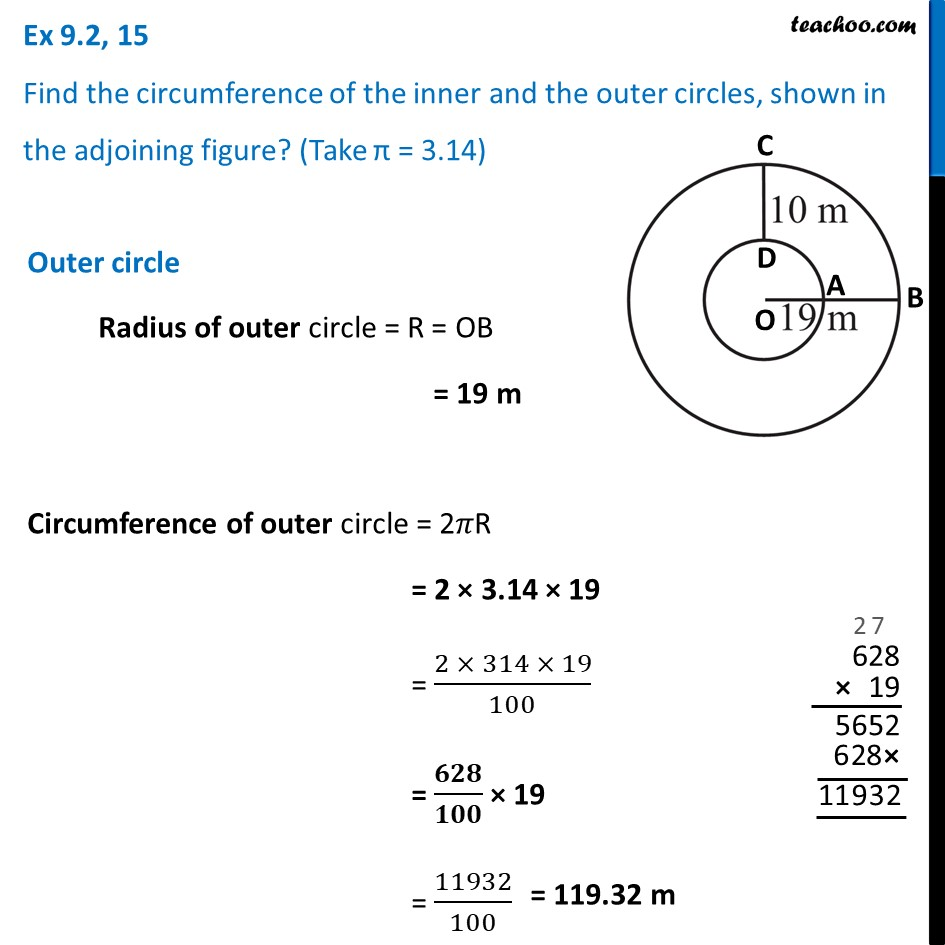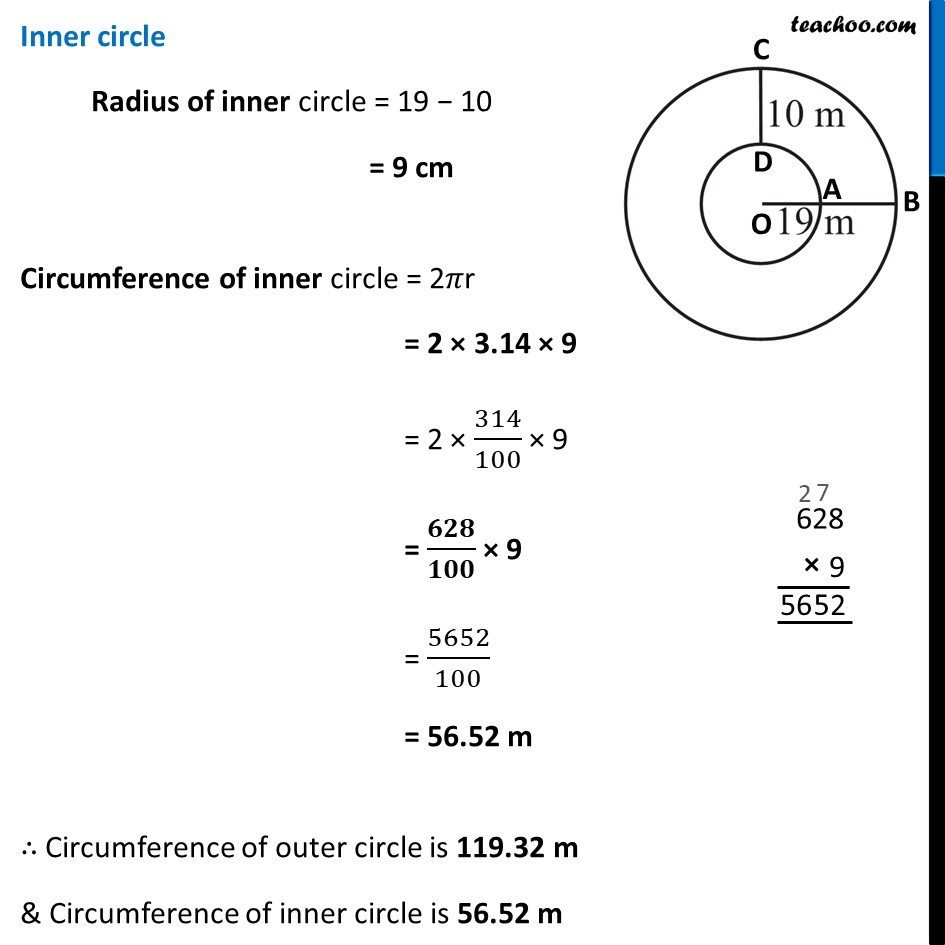Ex 9.2

Chapter 9 Class 7 Perimeter and Area
Serial order wiseLearn in your speed, with individual attention - Teachoo Maths 1-on-1 Class

### Transcript

Ex 9.2, 15 Find the circumference of the inner and the outer circles, shown in the adjoining figure? (Take π = 3.14) Outer circle Radius of outer circle = R = OB = 19 m Circumference of outer circle = 2𝜋R = 2 × 3.14 × 19 = (2 × 314 × 19)/100 = 𝟔𝟐𝟖/𝟏𝟎𝟎 × 19 = 11932/100 Inner circle Radius of inner circle = 19 − 10 = 9 cm Circumference of inner circle = 2𝜋r = 2 × 3.14 × 9 = 2 × 314/100 × 9 = 𝟔𝟐𝟖/𝟏𝟎𝟎 × 9 = 5652/100 = 56.52 m ∴ Circumference of outer circle is 119.32 m & Circumference of inner circle is 56.52 m